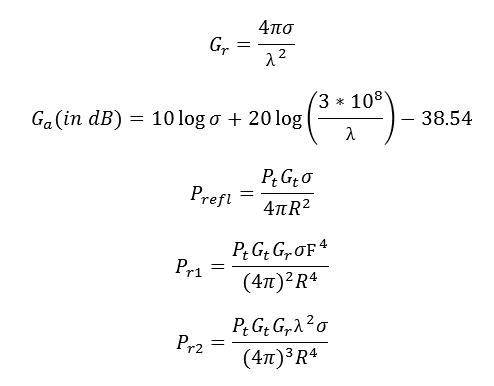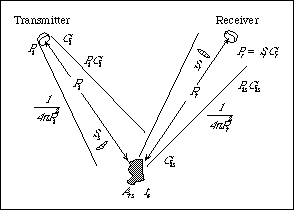# Radar Calculator

This CalcTown calculates the various parameters related to radio detection and ranging.

m2

#### Result

m2
dB
W
W
WClick here to view image

Where,

Pt = Transmitted Power

Pr1 = Power returning to the Receiver Antenna (1-way radar equation)

Pr2 = Power returning to the Receiver Antenna (2-way radar equation)

Prefl = Reflected signal from target

Gt = Gain of the Transmitting Antenna

Ga = Target Gain Factor or Gain of RCS

Gr = Effective Aperture of the Receiving Antenna

σ = Radar Cross-section or Scattering co-efficient of target

F = Pattern Propagation Factor

λ = Wavelength

R = Range (distance from transmitter or receiver to target)